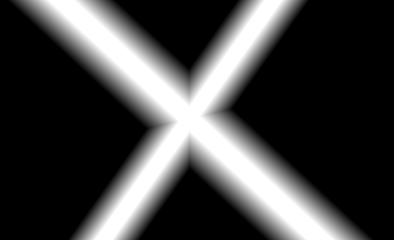# Instanced rendering alpha layering

I want to draw line using OpenGL instanced rendering.
Each line segment is an instance.
But OpenGL draws each segment as separated mesh, drawed using [glDrawArrays].
Because of this all segments are layered on top of each other.

How i can combine calls of GL functions ( glBlendFunc[Separate], glBlendEquation[Separate] ) to draw line segments on one alpha layer ( as one big mesh ) ?

screenshot : Pin on questions

So you want to avoid the double-rasterization and double-blending to some pixels rendered to by overlapping fragments?

There’s probably a lot of ways to accomplish this. But a few that come to mind:

1. Use the stencil buffer to avoid rasterizing to a pixel, or sample, multiple times (even though your primitives overlap), or
2. Switch to rasterizing area primitives (e.g. triangles) that don’t overlap.
1 Like

There are several ways to handle this, each with their drawbacks:

• Like DarkPhoton suggested: when drawing the things have the stencil test so that it tests for stencil == 0 and the stencil op to increment by 1. Then draw it again with color masking off with stencil == 1 and stencil op to decrement. I don’t like this because of the 2 passes.
• Render the content with no transparency to a texture then draw the texture with transparency. This is even worse than the first option because it uses up so much more bandwidth
• Set the depth test as ON and the depth test as GL_LESS (i.e. strict inequality) and have the vertex shader’s gl_Position.z the same (but not 1.0) for those draws. This will have the depth test do the occlusion for you. When drawing several groups of lines and you want to draw the overlap, then you need to feed a number to the vertex shader that gets decremented on each group. I usually like this way the most because it is likely nearly optimal for GPU’s since depth cull is a usually highly optimized and it does everything with a single state vector. The main downside is that it interacts quite poorly for doing 3D if the depth buffer is “busy”. In truth, I usually do this as setting the depth test as GL_GREATER, have an integer intZ in my drawing state that gets incremented on each “line group” where that Z value gets pushed to the vertex shader in some buffer (or just make it a uniform if you are ok with one draw per line group) and the vertex shader converts that integer intZ to a normalized depth value [-1, 1]. Depth buffer is usually 24-bits fixed, but gl_Position.z is fp32 so you have 22 or so bits you can definitely trust… so something like gl_Position.z = -1.0 + float(intZ) * constQ where Q is the constant 2.0f / (1u << 22u), where one trusts 22-bits. You can push more some, but 22 bits keep one safely away from precision errors with a 24-bit fixed or fp32 depth buffer.
1 Like

If you’re rasterizing convex polygons (as with the above image), you don’t need 2 passes. Just enable both stencil test and stencil write in the same pass, and never scribble on the same pixel or sample twice.

If OTOH you’re rasterizing concave polys (e.g. via triangle fans), that’s where you get into 2 passes:

• 1 to set the in/out counts in the stencil buffer (stencil write + no stencil test), and
• 1 to fill the “in polygon” pixels or samples (stencil test + no stencil write).
1 Like

In my application each line segment is a quad ( 4 vertices, drawed as GL_TRIANGLE_STRIP ).
In each fragment shader of segment quad I calculate the shortest distance between gl_FragCoord.xy and the line, determined by two points, transmitted from vertex shader.
By this distance i calculate antialiased border clip alpha value and multiply by the alpha of all segmented line.

``````float distance = lineDistance( gl_FragCoord.xy, v1, v2 );
float clipAlpha = smoothstep( thickness + 0.5, thickness - 0.5, distance );
color = vec4( 1.0, 0.5, 0.0, clipAlpha * lineAlpha );
``````

Therefore, unfortunately, i think, the depth test can’t help me with this problem.

You can `discard` the fragment if the alpha is zero. That just leaves the anti-aliased borders. If that’s an issue, you’ll have to render each layer separately and composite them.

1 Like

Can the next GL calls help me with this problem ?

``````glBlendFunc, glBlendEquation, glBlendFuncSeparate, glBlendEquationSeparate
``````

If your filled fragments were all either opaque or transparent, then depth test (or stencil test) could be easily used to solve your problem.

However, since your alpha values are translucent, I see your point. For a single pixel, essentially what you want is to:

• rasterize the fragment with the highest alpha value and
• kill the other one(s).

If you know you’ll be rendering on a constant background like this using the same line color (just different alpha values), you could probably get what you want by using a `GL_MAX` blend equation. See:

For an image of what this would look like, see:If those assumptions don’t work for you, you’ll need to use another approach, such as one of:

• Render these line segment quads with max blending to their own render target (fixed line color, constant BG color), and then composite that onto your other content.
• Render this group of lines segments with geometry that doesn’t occlude itself.
• Render the opaque core of your lines on one depth layer and the translucent joins/edges on another depth layer, and use DEPTH_TEST to ensure that the 100% opaque pixels always win (the join fades still need special care)
• etc.
1 Like

This topic was automatically closed 183 days after the last reply. New replies are no longer allowed.